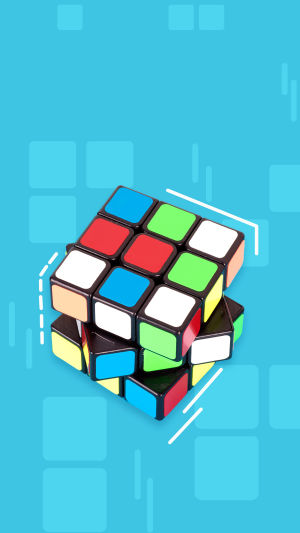The core of the Rubik's cube is three perpendicular axes, which ensure the smooth rotation of the cube.

In appearance, it consists of 26 small cubes to form a cube.

Including 6 central blocks connected with the central axis, fixed relative position, 12 edge blocks, there are two colors.

There are eight corner blocks with three colors.

In the restored state, each side of the cube is painted with the same color, and each of the six sides has a different color.

Each face of the cube can be turned freely, thus shuffling the cube and forming variable combinations.

Rubik's cube is divided into "positive order Rubik's cube" and "anomalous Rubik's cube" two types!

A positive order Rubik's cube is a cube.

According to the different order to name, there are second order, third order, up to 33 orders, special-shaped Rubik's cube, fan Rubik's cube, Zongzi Rubik's cube, mirror Rubik's cube, Maple leaf Rubik's cube, etc.

Once the Rubik's cube has been randomly disorganized, it is extremely difficult to restore it.

In recent years, many Rubik's cube players have invented many Rubik's cube plays, which can let a beginner learn to recover any arranged Rubik's cube in a short time.

The restoration methods include layer first method, Angle first method, edge first method, bridge method, CFOP method and so on.

One of the earliest and most popular methods is the layer-first method.

Rubik's cube recovery difficult, on the one hand is its upset after there are a large number of combinations, rubik's cube back another difficulty comes from.

We can only according to the specific way to recovery, repeated rotate one side, on the side of nine squares must be whole to participate in sports.

So that we will always disturb in the process of recovery is now part of, this limitation has greatly increased the difficulty of the rubik's cube.

Rubik's Cube is about developing the following abilities:

1. Vision

In the very beginning, there are many changes when playing the most basic third-order Rubik's cube, and the position of each Rubik's cube is observed and identified by eyes.

2. Hand strength

Turning Rubik's cube requires both hands, which can exercise the flexibility and coordination ability of hands well.

3. Memory

In the beginning of the recovery of the Rubik's cube, you need a good memory to remember some formulas, in order to improve their reduction speed, will be more practice.

4. Spatial judgment ability

When turning a Rubik's cube, it's always have to imagine how the cube can be restored.

In this process of imagination, we have exercised spatial judgment ability.

Recommend Pictures